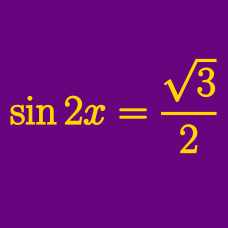Geometry

# Trigonometric Equations: Level 3 Challenges

Find the sum, in degrees, of all $\theta$ between $0^{\circ}$ and $360^{\circ}$ such that

$\tan(2\theta-330^{\circ})=\sqrt{3}.$

If $\sec x + \tan x = \dfrac{22}{7}$ and $\csc x + \cot x = \dfrac{m}{n}$, where $m$ and $n$ are coprime positive integers, find $m+n$.

The smallest positive solution of the equation $2 \sin^2 3x - \cos 8x - 1 = 0$ in the interval $\left( 0, \frac{\pi}{2}\right)$ can be expressed in the form $\frac{a\pi}{b}$, where $a$ and $b$ are coprime positive integers, find $a+b$.

The sum of all positive solutions of $2\cos 2x \left( \cos 2x- \cos\frac { 2014 \pi ^ 2 }{ x } \right) =\cos { 4x } -1$ is $k\pi$. Find $k$.

$\large \cos(p \cdot \sin x)=\sin(p \cdot \cos x)$ Find the smallest positive integer $p$ for which the above equation has a solution for $x \in [0, 2\pi]$.

×

Problem Loading...

Note Loading...

Set Loading...